# AP Physics C: Electricity and Magnetism Practice Test 5

### Test Information10 questions13 minutes

1.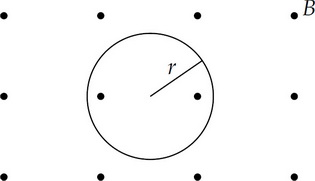A particle of charge –q and mass m moves with speed v perpendicular to a uniform magnetic field B directed out of the page. The path of the particle is a circle of radius r, as shown above.

The frequency with which the particle completes the circular path is

2. A 30 mF capacitor has 6 millicoulombs of charge on each plate. The energy stored in the capacitor is most nearly

3. Two large, parallel conducting plates have a potential difference of V maintained across them. A proton starts at rest on the surface of one plate and accelerates toward the other plate. Its acceleration in the region between the plates is proportional to

4.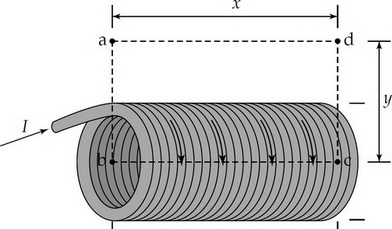An ideal solenoid with N total turns has a current I passing through the helical wires that make up the solenoid. Ampere's Law is used with a rectangular path abcd as shown above, to calculate the magnitude of the magnetic field B within the solenoid. The horizontal distances of the path are length x and the vertical distances of the path are length y. Which of the following equations results from the correct application of Ampere's law in this situation?

5.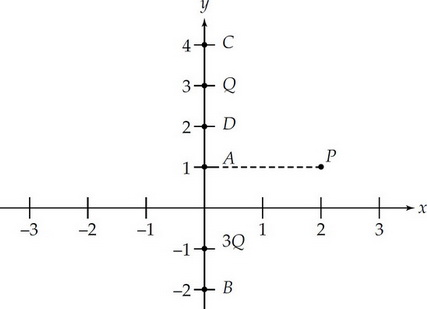Particles of charge +3Q and +Q are located on the y-axis as shown above. Assume the particles are isolated from other particles and are stationary. A, B, C, D, and P are points in the plane as indicated in the diagram.

Which of the following describes the direction of the electric field at point P?

6.Particles of charge +3Q and +Q are located on the y-axis as shown above. Assume the particles are isolated from other particles and are stationary. A, B, C, D, and P are points in the plane as indicated in the diagram.

At which of the labeled points is the electric potential zero?

7.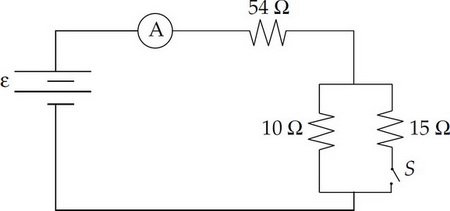When the switch S is closed in the circuit shown above the reading on the ammeter is 3 A. When the switch is opened the current through the 10 Ω resistor will

8. Two conducting cylindrical wires are made out of the same material. Wire X has twice the length and half the radius as wire Y. What is the ratio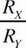of their resistances?

9.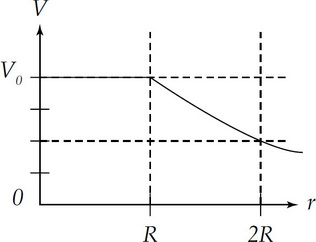A graph of electric potential V as a function of the radius from the origin r is shown above. What can be concluded about the electric field in the region 0 < r < R?

10. Two parallel wires, each carrying a current I, attract each other with a force F. If both currents are halved the attractive force is